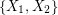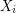# digraph

## Monochromatic reachability or rainbow triangles ★★★

Author(s): Sands; Sauer; Woodrow

In an edge-colored digraph, we say that a subgraph is rainbow if all its edges have distinct colors, and monochromatic if all its edges have the same color.

Problem   Letbe a tournament with edges colored from a set of three colors. Is it true thatmust have either a rainbow directed cycle of length three or a vertexso that every other vertex can be reached fromby a monochromatic (directed) path?

Keywords: digraph; edge-coloring; tournament

## Monochromatic reachability in edge-colored tournaments ★★★

Author(s): Erdos

Problem   For every, is there a (least) positive integerso that whenever a tournament has its edges colored withcolors, there exists a setof at mostvertices so that every vertex has a monochromatic path to some point in?

Keywords: digraph; edge-coloring; tournament

## Non-edges vs. feedback edge sets in digraphs ★★★

Author(s): Chudnovsky; Seymour; Sullivan

For any simple digraph, we letbe the number of unordered pairs of nonadjacent vertices (i.e. the number of non-edges), andbe the size of the smallest feedback edge set.

Conjecture  Ifis a simple digraph without directed cycles of length, then.

Keywords: acyclic; digraph; feedback edge set; triangle free

## Highly arc transitive two ended digraphs ★★

Author(s): Cameron; Praeger; Wormald

Conjecture   Ifis a highly arc transitive digraph with two ends, then every tile ofis a disjoint union of complete bipartite graphs.

Keywords: arc transitive; digraph; infinite graph

## Universal highly arc transitive digraphs ★★★

Author(s): Cameron; Praeger; Wormald

An alternating walk in a digraph is a walkso that the vertexis either the head of bothandor the tail of bothandfor every. A digraph is universal if for every pair of edges, there is an alternating walk containing bothandQuestion   Does there exist a locally finite highly arc transitive digraph which is universal?

Keywords: arc transitive; digraph

## Woodall's Conjecture ★★★

Author(s): Woodall

Conjecture   Ifis a directed graph with smallest directed cut of size, thenhasdisjoint dijoins.

Keywords: digraph; packing

## The Two Color Conjecture ★★

Author(s): Neumann-Lara

Conjecture   Ifis an orientation of a simple planar graph, then there is a partition ofintoso that the graph induced byis acyclic for.

Keywords: acyclic; digraph; planar